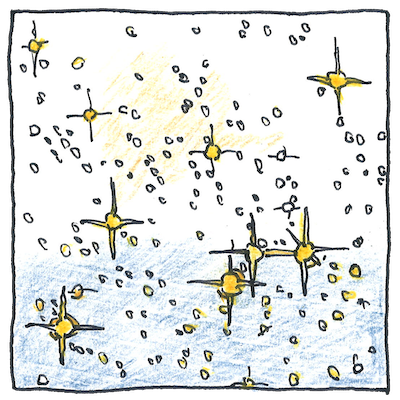# 1971

## The book of science

Tom Sharp

 Hannes Alfvén, Oskar Klein cosmology

## Plasma cosmology

• With regard to the formation
• of planets, stars, galaxies,
• and the universe,
• plasma permeates the cosmos
• so let’s not assume
• only ordinary matter matters.
• *
• Does electromagnetism in plasmas
• affect charged particles in space
• more than gravity?
• Could the theory of double layers
• explain baryon asymmetry
• and the origin of our universe?
• *
• In the cosmology
• of Alfvén and Klein,
• we live in an ambiplasma bubble
• that has expanded beyond
• the visible universe.

## Plasma scaling

• The secret of scaling
• plasma parameters
• are questionable laws
• without dimensions.
• Plasma equations have many factors—
• capacitance, conductivity, current,
• current density, electric field,
• electron density, gas density,
• inductance, ion density,
• ionization fraction, length,
• magnetic field, particle energy,
• potential, resistance, time, and velocity—
• all related to each other.
• Ignoring inessentials
• that respect similarities
• across orders of magnitude.

## Vast

• Big. Really big.
• More than are dreamt of.